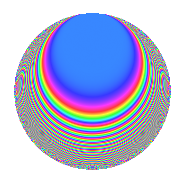# Properties

 Label 900.1.bhLevel 900 Weight 1 Character orbit bh Rep. character $$\chi_{900}(287,\cdot)$$ Character field $$\Q(\zeta_{20})$$ Dimension 16 Newforms 1 Sturm bound 180 Trace bound 0

# Related objects

## Defining parameters

 Level: $$N$$ = $$900 = 2^{2} \cdot 3^{2} \cdot 5^{2}$$ Weight: $$k$$ = $$1$$ Character orbit: $$[\chi]$$ = 900.bh (of order $$20$$ and degree $$8$$) Character conductor: $$\operatorname{cond}(\chi)$$ = $$300$$ Character field: $$\Q(\zeta_{20})$$ Newforms: $$1$$ Sturm bound: $$180$$ Trace bound: $$0$$

## Dimensions

The following table gives the dimensions of various subspaces of $$M_{1}(900, [\chi])$$.

Total New Old
Modular forms 80 16 64
Cusp forms 16 16 0
Eisenstein series 64 0 64

The following table gives the dimensions of subspaces with specified projective image type.

$$D_n$$ $$A_4$$ $$S_4$$ $$A_5$$
Dimension 16 0 0 0

## Trace form

 $$16q + O(q^{10})$$ $$16q + 4q^{13} + 4q^{16} - 20q^{34} + 4q^{37} - 4q^{40} - 4q^{52} - 4q^{58} - 4q^{73} + 4q^{82} - 20q^{85} - 4q^{97} + O(q^{100})$$

## Decomposition of $$S_{1}^{\mathrm{new}}(900, [\chi])$$ into irreducible Hecke orbits

Label Dim. $$A$$ Field Image CM RM Traces $q$-expansion
$$a_2$$ $$a_3$$ $$a_5$$ $$a_7$$
900.1.bh.a $$16$$ $$0.449$$ $$\Q(\zeta_{40})$$ $$D_{20}$$ $$\Q(\sqrt{-1})$$ None $$0$$ $$0$$ $$0$$ $$0$$ $$q-\zeta_{40}^{11}q^{2}-\zeta_{40}^{2}q^{4}+\zeta_{40}^{3}q^{5}+\cdots$$